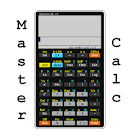MC40 Scientific Calculator

All Android applications categories

All Android games categories# MC40 Scientific Calculator

662 8.2

8.2 Users
rating

## Screenshots

Description

Features:
-Complete User's guide. (available also in Spanish)

-Two keyboard styles.
-Degrees, Radians, Scientific and Engineering notation, fixed number of decimals and floating point, sexagesimal system.

-Complex numbers, fractions

-Implied multiplication.

-Retrieve the last 30 results and math expressions.

-Built-in functions:
Logarithms, trigonometric and hyperbolic functions, factorial, combinations, permutations, greatest common divisor, least common multiple, absolute value, modulus, complex numbers, prime and random numbers, determinants, vectors ( norm, dot product).

-Store results in memories and/or user defined variables.

-21 built-in constants.
-Unit converter: 120 predefined Measurement Units.

-User defined functions and constants.
-User defined formulas: Evaluate automatically your own formulas.

-Greek alphabet.

-Functions and derivatives graphing.(up to 3 functions). Tables.
-Zeros of functions, maxima and minima.

- Matrix operations (addition, subtraction, multiplication, transpose, inverse).
-Solve directly systems of linear equations up to a size of 7 x 7 using Cramer's rule.

-Vector operations (addition, subtraction, cross product).

-Statistical Calculations: mean, standard deviation, linear regression.

This app includes ads. If you prefer you can also install the paid version (model MC50) without ads.

from 662 reviews

"Great"

8.2